Main MRPT website > C++ reference for MRPT 1.5.6
mrpt::poses::CPosePDFSOG Class Referenceabstract

Detailed Description

Declares a class that represents a Probability Density function (PDF) of a 2D pose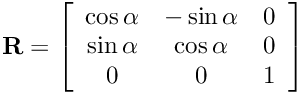.

This class implements that PDF as the following multi-modal Gaussian distribution: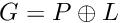Where the number of modes N is the size of CPosePDFSOG::m_modes

See mrpt::poses::CPosePDF for more details.

CPose2D, CPosePDF, CPosePDFParticles

Definition at line 37 of file CPosePDFSOG.h.

#include <mrpt/poses/CPosePDFSOG.h>

Inheritance diagram for mrpt::poses::CPosePDFSOG: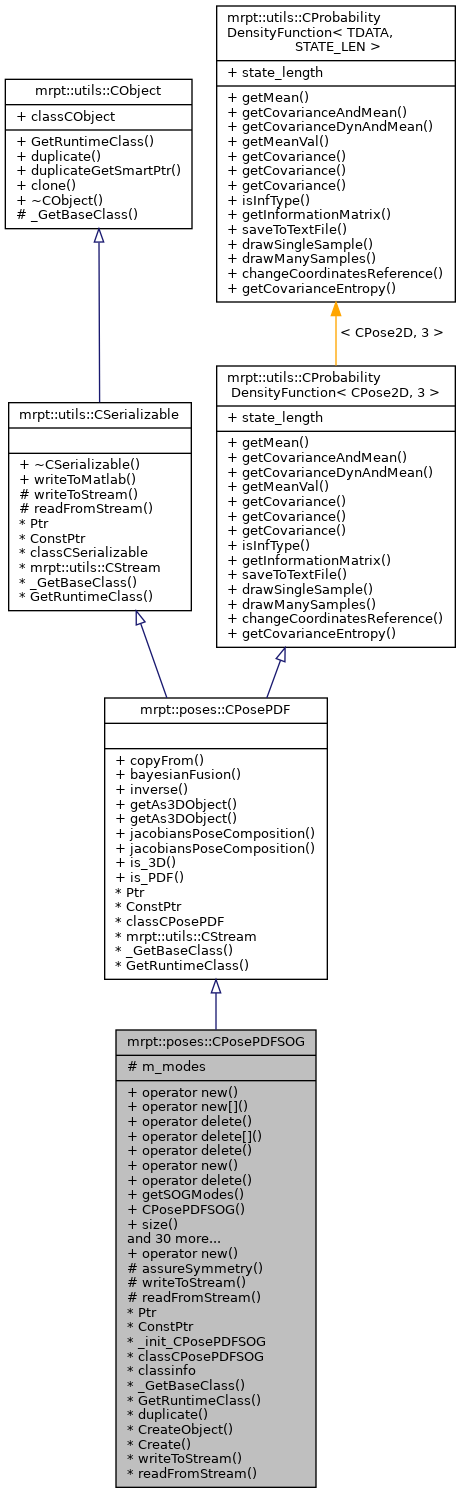Classes

struct  TGaussianMode
The struct for each mode: More...

Public Types

typedef
mrpt::aligned_containers
< TGaussianMode >::vector_t
CListGaussianModes

typedef
CListGaussianModes::const_iterator
const_iterator

typedef
CListGaussianModes::iterator
iterator

enum  { is_3D_val = 0 }

enum  { is_PDF_val = 1 }

typedef CPose2D type_value
The type of the state the PDF represents. More...

typedef
CProbabilityDensityFunction
< CPose2D, STATE_LEN >
self_t

Public Member Functions

voidoperator new (size_t size)

voidoperator new[] (size_t size)

void operator delete (void *ptr) throw ()

void operator delete[] (void *ptr) throw ()

void operator delete (void *memory, void *ptr) throw ()

voidoperator new (size_t size, const std::nothrow_t &) throw ()

void operator delete (void *ptr, const std::nothrow_t &) throw ()

const CListGaussianModesgetSOGModes () const

CPosePDFSOG (size_t nModes=1)
Default constructor. More...

size_t size () const
Return the number of Gaussian modes. More...

bool empty () const
Return whether there is any Gaussian mode. More...

void clear ()
Clear the list of modes. More...

const TGaussianModeoperator[] (size_t i) const

TGaussianModeoperator[] (size_t i)

const TGaussianModeget (size_t i) const

TGaussianModeget (size_t i)

void push_back (const TGaussianMode &m)
Inserts a copy of the given mode into the SOG. More...

iterator begin ()

iterator end ()

const_iterator begin () const

const_iterator end () const

iterator erase (iterator i)

void resize (const size_t N)
Resize the number of SOG modes. More...

void mergeModes (double max_KLd=0.5, bool verbose=false)
Merge very close modes so the overall number of modes is reduced while preserving the total distribution. More...

void getMean (CPose2D &mean_pose) const MRPT_OVERRIDE
Returns an estimate of the pose, (the mean, or mathematical expectation of the PDF) More...

void getCovarianceAndMean (mrpt::math::CMatrixDouble33 &cov, CPose2D &mean_point) const MRPT_OVERRIDE
Returns an estimate of the pose covariance matrix (3x3 cov matrix) and the mean, both at once. More...

void getMostLikelyCovarianceAndMean (mrpt::math::CMatrixDouble33 &cov, CPose2D &mean_point) const
For the most likely Gaussian mode in the SOG, returns the pose covariance matrix (3x3 cov matrix) and the mean. More...

void normalizeWeights ()
Normalize the weights in m_modes such as the maximum log-weight is 0. More...

void copyFrom (const CPosePDF &o) MRPT_OVERRIDE
Copy operator, translating if necesary (for example, between particles and gaussian representations) More...

void saveToTextFile (const std::string &file) const MRPT_OVERRIDE
Save the density to a text file, with the following format: There is one row per Gaussian "mode", and each row contains 10 elements: More...

void changeCoordinatesReference (const CPose3D &newReferenceBase) MRPT_OVERRIDE
this = p (+) this. More...

void rotateAllCovariances (const double &ang)
Rotate all the covariance matrixes by replacing them by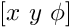, where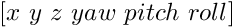. More...

void drawSingleSample (CPose2D &outPart) const MRPT_OVERRIDE
Draws a single sample from the distribution. More...

void drawManySamples (size_t N, std::vector< mrpt::math::CVectorDouble > &outSamples) const MRPT_OVERRIDE
Draws a number of samples from the distribution, and saves as a list of 1x3 vectors, where each row contains a (x,y,phi) datum. More...

void inverse (CPosePDF &o) const MRPT_OVERRIDE
Returns a new PDF such as: NEW_PDF = (0,0,0) - THIS_PDF. More...

void operator+= (const mrpt::poses::CPose2D &Ap)
Makes: thisPDF = thisPDF + Ap, where "+" is pose composition (both the mean, and the covariance matrix are updated). More...

double evaluatePDF (const mrpt::poses::CPose2D &x, bool sumOverAllPhis=false) const
Evaluates the PDF at a given point. More...

double evaluateNormalizedPDF (const mrpt::poses::CPose2D &x) const
Evaluates the ratio PDF(x) / max_PDF(x*), that is, the normalized PDF in the range [0,1]. More...

void evaluatePDFInArea (const double &x_min, const double &x_max, const double &y_min, const double &y_max, const double &resolutionXY, const double &phi, mrpt::math::CMatrixD &outMatrix, bool sumOverAllPhis=false)
Evaluates the PDF within a rectangular grid (and a fixed orientation) and saves the result in a matrix (each row contains values for a fixed y-coordinate value). More...

void bayesianFusion (const CPosePDF &p1, const CPosePDF &p2, const double &minMahalanobisDistToDrop=0) MRPT_OVERRIDE
Bayesian fusion of two pose distributions, then save the result in this object (WARNING: Currently p1 must be a mrpt::poses::CPosePDFSOG object and p2 a mrpt::poses::CPosePDFGaussian object) More...

template<class OPENGL_SETOFOBJECTSPTR >
void getAs3DObject (OPENGL_SETOFOBJECTSPTR &out_obj) const
Returns a 3D representation of this PDF (it doesn't clear the current contents of out_obj, but append new OpenGL objects to that list) More...

template<class OPENGL_SETOFOBJECTSPTR >
OPENGL_SETOFOBJECTSPTR getAs3DObject () const
Returns a 3D representation of this PDF. More...

virtual mxArraywriteToMatlab () const
Introduces a pure virtual method responsible for writing to a mxArray Matlab object, typically a MATLAB struct whose contents are documented in each derived class. More...

mrpt::utils::CObjectPtr duplicateGetSmartPtr () const
Returns a copy of the object, indepently of its class, as a smart pointer (the newly created object will exist as long as any copy of this smart pointer). More...

CObjectclone () const
Cloning interface for smart pointers. More...

virtual void getMean (CPose2D &mean_point) const =0
Returns the mean, or mathematical expectation of the probability density distribution (PDF). More...

virtual void getCovarianceAndMean (mrpt::math::CMatrixFixedNumeric< double, STATE_LEN, STATE_LEN > &cov, CPose2D &mean_point) const =0
Returns an estimate of the pose covariance matrix (STATE_LENxSTATE_LEN cov matrix) and the mean, both at once. More...

void getCovarianceDynAndMean (mrpt::math::CMatrixDouble &cov, CPose2D &mean_point) const
Returns an estimate of the pose covariance matrix (STATE_LENxSTATE_LEN cov matrix) and the mean, both at once. More...

CPose2D getMeanVal () const
Returns the mean, or mathematical expectation of the probability density distribution (PDF). More...

void getCovariance (mrpt::math::CMatrixDouble &cov) const
Returns the estimate of the covariance matrix (STATE_LEN x STATE_LEN covariance matrix) More...

void getCovariance (mrpt::math::CMatrixFixedNumeric< double, STATE_LEN, STATE_LEN > &cov) const
Returns the estimate of the covariance matrix (STATE_LEN x STATE_LEN covariance matrix) More...

mrpt::math::CMatrixFixedNumeric
< double, STATE_LEN, STATE_LEN >
getCovariance () const
Returns the estimate of the covariance matrix (STATE_LEN x STATE_LEN covariance matrix) More...

virtual bool isInfType () const
Returns whether the class instance holds the uncertainty in covariance or information form. More...

virtual void getInformationMatrix (mrpt::math::CMatrixFixedNumeric< double, STATE_LEN, STATE_LEN > &inf) const
Returns the information (inverse covariance) matrix (a STATE_LEN x STATE_LEN matrix) Unless reimplemented in derived classes, this method first reads the covariance, then invert it. More...

virtual void drawSingleSample (CPose2D &outPart) const =0
Draws a single sample from the distribution. More...

double getCovarianceEntropy () const
Compute the entropy of the estimated covariance matrix. More...

Static Public Member Functions

static voidoperator new (size_t size, void *ptr)

static void jacobiansPoseComposition (const CPose2D &x, const CPose2D &u, mrpt::math::CMatrixDouble33 &df_dx, mrpt::math::CMatrixDouble33 &df_du, const bool compute_df_dx=true, const bool compute_df_du=true)
This static method computes the pose composition Jacobians, with these formulas: More...

static void jacobiansPoseComposition (const CPosePDFGaussian &x, const CPosePDFGaussian &u, mrpt::math::CMatrixDouble33 &df_dx, mrpt::math::CMatrixDouble33 &df_du)

static bool is_3D ()

static bool is_PDF ()

Static Public Attributes

static const
mrpt::utils::TRuntimeClassId
classCObject

static const size_t state_length
The length of the variable, for example, 3 for a 3D point, 6 for a 3D pose (x y z yaw pitch roll). More...

RTTI stuff
static const
mrpt::utils::TRuntimeClassId
classCPosePDF

RTTI stuff
static const
mrpt::utils::TRuntimeClassId
classCSerializable

Protected Member Functions

void assureSymmetry ()
Ensures the symmetry of the covariance matrix (eventually certain operations in the math-coprocessor lead to non-symmetric matrixes!) More...

CSerializable virtual methods
void writeToStream (mrpt::utils::CStream &out, int *getVersion) const
Introduces a pure virtual method responsible for writing to a CStream. More...

void readFromStream (mrpt::utils::CStream &in, int version)
Introduces a pure virtual method responsible for loading from a CStream This can not be used directly be users, instead use "stream >> object;" for reading it from a stream or "stream >> object_ptr;" if the class is unknown apriori. More...

Protected Attributes

CListGaussianModes m_modes
The list of SOG modes. More...

RTTI stuff

typedef CPosePDFSOGPtr Ptr

typedef CPosePDFSOGPtr ConstPtr

static mrpt::utils::CLASSINIT _init_CPosePDFSOG

static mrpt::utils::TRuntimeClassId classCPosePDFSOG

static const
mrpt::utils::TRuntimeClassId
classinfo

static const
mrpt::utils::TRuntimeClassId
_GetBaseClass ()

virtual const
mrpt::utils::TRuntimeClassId
GetRuntimeClass () const
Returns information about the class of an object in runtime. More...

virtual mrpt::utils::CObjectduplicate () const
Returns a copy of the object, indepently of its class. More...

static mrpt::utils::CObjectCreateObject ()

static CPosePDFSOGPtr Create ()

Member Typedef Documentation

 typedef mrpt::aligned_containers::vector_t mrpt::poses::CPosePDFSOG::CListGaussianModes

Definition at line 71 of file CPosePDFSOG.h.

 typedef CListGaussianModes::const_iterator mrpt::poses::CPosePDFSOG::const_iterator

Definition at line 72 of file CPosePDFSOG.h.

 typedef CPosePDFSOGPtr mrpt::poses::CPosePDFSOG::ConstPtr

Definition at line 40 of file CPosePDFSOG.h.

 typedef CListGaussianModes::iterator mrpt::poses::CPosePDFSOG::iterator

Definition at line 73 of file CPosePDFSOG.h.

 typedef CPosePDFSOGPtr mrpt::poses::CPosePDFSOG::Ptr

A typedef for the associated smart pointer

Definition at line 40 of file CPosePDFSOG.h.

 typedef CProbabilityDensityFunction mrpt::utils::CProbabilityDensityFunction< CPose2D , STATE_LEN >::self_t
inherited

Definition at line 33 of file CProbabilityDensityFunction.h.

 typedef CPose2D mrpt::utils::CProbabilityDensityFunction< CPose2D , STATE_LEN >::type_value
inherited

The type of the state the PDF represents.

Definition at line 32 of file CProbabilityDensityFunction.h.

Member Enumeration Documentation

 anonymous enum
inherited
Enumerator
is_3D_val

Definition at line 91 of file CPosePDF.h.

 anonymous enum
inherited
Enumerator
is_PDF_val

Definition at line 93 of file CPosePDF.h.

Constructor & Destructor Documentation

 CPosePDFSOG::CPosePDFSOG ( size_t nModes = 1 )

Default constructor.

Parameters
 nModes The initial size of CPosePDFSOG::m_modes

Definition at line 36 of file CPosePDFSOG.cpp.

Member Function Documentation

 static const mrpt::utils::TRuntimeClassId* mrpt::poses::CPosePDFSOG::_GetBaseClass ( )
staticprotected
 void CPosePDFSOG::assureSymmetry ( )
protected

Ensures the symmetry of the covariance matrix (eventually certain operations in the math-coprocessor lead to non-symmetric matrixes!)

Definition at line 491 of file CPosePDFSOG.cpp.

References mrpt::math::cov().

 void CPosePDFSOG::bayesianFusion ( const CPosePDF & p1, const CPosePDF & p2, const double & minMahalanobisDistToDrop = 0 )
virtual

Bayesian fusion of two pose distributions, then save the result in this object (WARNING: Currently p1 must be a mrpt::poses::CPosePDFSOG object and p2 a mrpt::poses::CPosePDFGaussian object)

Implements mrpt::poses::CPosePDF.

Definition at line 315 of file CPosePDFSOG.cpp.

 iterator mrpt::poses::CPosePDFSOG::begin ( )
inline

Definition at line 120 of file CPosePDFSOG.h.

 const_iterator mrpt::poses::CPosePDFSOG::begin ( ) const
inline

Definition at line 122 of file CPosePDFSOG.h.

 void CPosePDFSOG::changeCoordinatesReference ( const CPose3D & newReferenceBase )
virtual

this = p (+) this.

This can be used to convert a PDF from local coordinates to global, providing the point (newReferenceBase) from which "to project" the current pdf. Result PDF substituted the currently stored one in the object.

Definition at line 239 of file CPosePDFSOG.cpp.

References mrpt::math::cov(), mrpt::poses::CPose2D::getHomogeneousMatrix(), and mean().

 void CPosePDFSOG::clear ( )

Clear the list of modes.

Definition at line 43 of file CPosePDFSOG.cpp.

Referenced by mrpt::tfest::se2_l2_robust().

 CObject* mrpt::utils::CObject::clone ( ) const
inlineinherited

Cloning interface for smart pointers.

Definition at line 143 of file CObject.h.

 void CPosePDFSOG::copyFrom ( const CPosePDF & o )
virtual

Copy operator, translating if necesary (for example, between particles and gaussian representations)

Implements mrpt::poses::CPosePDF.

Definition at line 196 of file CPosePDFSOG.cpp.

 static CPosePDFSOGPtr mrpt::poses::CPosePDFSOG::Create ( )
static
 static mrpt::utils::CObject* mrpt::poses::CPosePDFSOG::CreateObject ( )
static
 void CPosePDFSOG::drawManySamples ( size_t N, std::vector< mrpt::math::CVectorDouble > & outSamples ) const
virtual

Draws a number of samples from the distribution, and saves as a list of 1x3 vectors, where each row contains a (x,y,phi) datum.

Reimplemented from mrpt::utils::CProbabilityDensityFunction< CPose2D, 3 >.

Definition at line 298 of file CPosePDFSOG.cpp.

References MRPT_END, MRPT_START, MRPT_UNUSED_PARAM, and THROW_EXCEPTION.

 virtual void mrpt::utils::CProbabilityDensityFunction< CPose2D , STATE_LEN >::drawSingleSample ( CPose2D & outPart ) const
pure virtualinherited

Draws a single sample from the distribution.

 void CPosePDFSOG::drawSingleSample ( CPose2D & outPart ) const

Draws a single sample from the distribution.

Definition at line 285 of file CPosePDFSOG.cpp.

References MRPT_END, MRPT_START, MRPT_UNUSED_PARAM, and THROW_EXCEPTION.

 virtual mrpt::utils::CObject* mrpt::poses::CPosePDFSOG::duplicate ( ) const
virtual

Returns a copy of the object, indepently of its class.

Implements mrpt::utils::CObject.

 mrpt::utils::CObjectPtr mrpt::utils::CObject::duplicateGetSmartPtr ( ) const
inlineinherited

Returns a copy of the object, indepently of its class, as a smart pointer (the newly created object will exist as long as any copy of this smart pointer).

Definition at line 140 of file CObject.h.

 bool mrpt::poses::CPosePDFSOG::empty ( ) const
inline

Return whether there is any Gaussian mode.

Definition at line 88 of file CPosePDFSOG.h.

 iterator mrpt::poses::CPosePDFSOG::end ( )
inline

Definition at line 121 of file CPosePDFSOG.h.

 const_iterator mrpt::poses::CPosePDFSOG::end ( ) const
inline

Definition at line 123 of file CPosePDFSOG.h.

 iterator mrpt::poses::CPosePDFSOG::erase ( iterator i )
inline

Definition at line 125 of file CPosePDFSOG.h.

 double CPosePDFSOG::evaluateNormalizedPDF ( const mrpt::poses::CPose2D & x ) const

Evaluates the ratio PDF(x) / max_PDF(x*), that is, the normalized PDF in the range [0,1].

Definition at line 473 of file CPosePDFSOG.cpp.

References mrpt::math::cov(), mean(), and mrpt::math::normalPDF().

 double CPosePDFSOG::evaluatePDF ( const mrpt::poses::CPose2D & x, bool sumOverAllPhis = false ) const

Evaluates the PDF at a given point.

Definition at line 426 of file CPosePDFSOG.cpp.

 void CPosePDFSOG::evaluatePDFInArea ( const double & x_min, const double & x_max, const double & y_min, const double & y_max, const double & resolutionXY, const double & phi, mrpt::math::CMatrixD & outMatrix, bool sumOverAllPhis = false )

Evaluates the PDF within a rectangular grid (and a fixed orientation) and saves the result in a matrix (each row contains values for a fixed y-coordinate value).

Definition at line 525 of file CPosePDFSOG.cpp.

References ASSERT_, MRPT_END, and MRPT_START.

 const TGaussianMode& mrpt::poses::CPosePDFSOG::get ( size_t i ) const
inline

Definition at line 105 of file CPosePDFSOG.h.

References ASSERT_.

Referenced by mrpt::tfest::se2_l2_robust().

 TGaussianMode& mrpt::poses::CPosePDFSOG::get ( size_t i )
inline

Definition at line 110 of file CPosePDFSOG.h.

References ASSERT_.

template<class OPENGL_SETOFOBJECTSPTR >
 void mrpt::poses::CPosePDF::getAs3DObject ( OPENGL_SETOFOBJECTSPTR & out_obj ) const
inlineinherited

Returns a 3D representation of this PDF (it doesn't clear the current contents of out_obj, but append new OpenGL objects to that list)

Note
Needs the mrpt-opengl library, and using mrpt::opengl::CSetOfObjectsPtr as template argument.
By default, ellipsoids for the confidence intervals of "q=3" are drawn; for more mathematical details, see CGeneralizedEllipsoidTemplate::setQuantiles()

Definition at line 101 of file CPosePDF.h.

References mrpt::opengl::posePDF2opengl().

template<class OPENGL_SETOFOBJECTSPTR >
 OPENGL_SETOFOBJECTSPTR mrpt::poses::CPosePDF::getAs3DObject ( ) const
inlineinherited

Returns a 3D representation of this PDF.

Note
Needs the mrpt-opengl library, and using mrpt::opengl::CSetOfObjectsPtr as template argument.

Definition at line 110 of file CPosePDF.h.

References mrpt::opengl::posePDF2opengl().

 void mrpt::utils::CProbabilityDensityFunction< CPose2D , STATE_LEN >::getCovariance ( mrpt::math::CMatrixDouble & cov ) const
inlineinherited

Returns the estimate of the covariance matrix (STATE_LEN x STATE_LEN covariance matrix)

getMean, getCovarianceAndMean, getInformationMatrix

Definition at line 68 of file CProbabilityDensityFunction.h.

 void mrpt::utils::CProbabilityDensityFunction< CPose2D , STATE_LEN >::getCovariance ( mrpt::math::CMatrixFixedNumeric< double, STATE_LEN, STATE_LEN > & cov ) const
inlineinherited

Returns the estimate of the covariance matrix (STATE_LEN x STATE_LEN covariance matrix)

getMean, getCovarianceAndMean, getInformationMatrix

Definition at line 77 of file CProbabilityDensityFunction.h.

 mrpt::math::CMatrixFixedNumeric mrpt::utils::CProbabilityDensityFunction< CPose2D , STATE_LEN >::getCovariance ( ) const
inlineinherited

Returns the estimate of the covariance matrix (STATE_LEN x STATE_LEN covariance matrix)

getMean, getInformationMatrix

Definition at line 86 of file CProbabilityDensityFunction.h.

 virtual void mrpt::utils::CProbabilityDensityFunction< CPose2D , STATE_LEN >::getCovarianceAndMean ( mrpt::math::CMatrixFixedNumeric< double, STATE_LEN, STATE_LEN > & cov, CPose2D & mean_point ) const
pure virtualinherited

Returns an estimate of the pose covariance matrix (STATE_LENxSTATE_LEN cov matrix) and the mean, both at once.

getMean, getInformationMatrix
 void CPosePDFSOG::getCovarianceAndMean ( mrpt::math::CMatrixDouble33 & cov, CPose2D & mean_point ) const

Returns an estimate of the pose covariance matrix (3x3 cov matrix) and the mean, both at once.

getMean

Definition at line 81 of file CPosePDFSOG.cpp.

References mrpt::math::cov(), and mean().

 void mrpt::utils::CProbabilityDensityFunction< CPose2D , STATE_LEN >::getCovarianceDynAndMean ( mrpt::math::CMatrixDouble & cov, CPose2D & mean_point ) const
inlineinherited

Returns an estimate of the pose covariance matrix (STATE_LENxSTATE_LEN cov matrix) and the mean, both at once.

getMean, getInformationMatrix

Definition at line 48 of file CProbabilityDensityFunction.h.

 double mrpt::utils::CProbabilityDensityFunction< CPose2D , STATE_LEN >::getCovarianceEntropy ( ) const
inlineinherited

Compute the entropy of the estimated covariance matrix.

http://en.wikipedia.org/wiki/Multivariate_normal_distribution#Entropy

Definition at line 144 of file CProbabilityDensityFunction.h.

 virtual void mrpt::utils::CProbabilityDensityFunction< CPose2D , STATE_LEN >::getInformationMatrix ( mrpt::math::CMatrixFixedNumeric< double, STATE_LEN, STATE_LEN > & inf ) const
inlinevirtualinherited

Returns the information (inverse covariance) matrix (a STATE_LEN x STATE_LEN matrix) Unless reimplemented in derived classes, this method first reads the covariance, then invert it.

getMean, getCovarianceAndMean

Definition at line 106 of file CProbabilityDensityFunction.h.

 virtual void mrpt::utils::CProbabilityDensityFunction< CPose2D , STATE_LEN >::getMean ( CPose2D & mean_point ) const
pure virtualinherited

Returns the mean, or mathematical expectation of the probability density distribution (PDF).

getCovarianceAndMean, getInformationMatrix
 void CPosePDFSOG::getMean ( CPose2D & mean_pose ) const

Returns an estimate of the pose, (the mean, or mathematical expectation of the PDF)

getCovariance

Definition at line 61 of file CPosePDFSOG.cpp.

 CPose2D mrpt::utils::CProbabilityDensityFunction< CPose2D , STATE_LEN >::getMeanVal ( ) const
inlineinherited

Returns the mean, or mathematical expectation of the probability density distribution (PDF).

getCovariance, getInformationMatrix

Definition at line 58 of file CProbabilityDensityFunction.h.

 void CPosePDFSOG::getMostLikelyCovarianceAndMean ( mrpt::math::CMatrixDouble33 & cov, CPose2D & mean_point ) const

For the most likely Gaussian mode in the SOG, returns the pose covariance matrix (3x3 cov matrix) and the mean.

getMean

Definition at line 687 of file CPosePDFSOG.cpp.

References begin().

 virtual const mrpt::utils::TRuntimeClassId* mrpt::poses::CPosePDFSOG::GetRuntimeClass ( ) const
virtual

Returns information about the class of an object in runtime.

Reimplemented from mrpt::poses::CPosePDF.

 const CListGaussianModes& mrpt::poses::CPosePDFSOG::getSOGModes ( ) const
inline

Definition at line 75 of file CPosePDFSOG.h.

 void CPosePDFSOG::inverse ( CPosePDF & o ) const
virtual

Returns a new PDF such as: NEW_PDF = (0,0,0) - THIS_PDF.

Implements mrpt::poses::CPosePDF.

Definition at line 391 of file CPosePDFSOG.cpp.

 static bool mrpt::poses::CPosePDF::is_3D ( )
inlinestaticinherited

Definition at line 92 of file CPosePDF.h.

 static bool mrpt::poses::CPosePDF::is_PDF ( )
inlinestaticinherited

Definition at line 94 of file CPosePDF.h.

 virtual bool mrpt::utils::CProbabilityDensityFunction< CPose2D , STATE_LEN >::isInfType ( ) const
inlinevirtualinherited

Returns whether the class instance holds the uncertainty in covariance or information form.

Note
By default this is going to be covariance form. *Inf classes (e.g. CPosePDFGaussianInf) store it in information form.
mrpt::traits::is_inf_type

Reimplemented in mrpt::poses::CPosePDFGaussianInf.

Definition at line 100 of file CProbabilityDensityFunction.h.

 void CPosePDF::jacobiansPoseComposition ( const CPose2D & x, const CPose2D & u, mrpt::math::CMatrixDouble33 & df_dx, mrpt::math::CMatrixDouble33 & df_du, const bool compute_df_dx = true, const bool compute_df_du = true )
staticinherited

This static method computes the pose composition Jacobians, with these formulas:

df_dx =
[ 1, 0, -sin(phi_x)*x_u-cos(phi_x)*y_u ]
[ 0, 1, cos(phi_x)*x_u-sin(phi_x)*y_u ]
[ 0, 0, 1 ]
df_du =
[ cos(phi_x) , -sin(phi_x) , 0 ]
[ sin(phi_x) , cos(phi_x) , 0 ]
[ 0 , 0 , 1 ]

Definition at line 35 of file CPosePDF.cpp.

 void CPosePDF::jacobiansPoseComposition ( const CPosePDFGaussian & x, const CPosePDFGaussian & u, mrpt::math::CMatrixDouble33 & df_dx, mrpt::math::CMatrixDouble33 & df_du )
staticinherited

This is an overloaded member function, provided for convenience. It differs from the above function only in what argument(s) it accepts.

Definition at line 23 of file CPosePDF.cpp.

 void CPosePDFSOG::mergeModes ( double max_KLd = 0.5, bool verbose = false )

Merge very close modes so the overall number of modes is reduced while preserving the total distribution.

This method uses the approach described in the paper:

• "Kullback-Leibler Approach to Gaussian Mixture Reduction" AR Runnalls. IEEE Transactions on Aerospace and Electronic Systems, 2007.
Parameters
 max_KLd The maximum KL-divergence to consider the merge of two nodes (and then stops the process).

Definition at line 563 of file CPosePDFSOG.cpp.

 void CPosePDFSOG::normalizeWeights ( )

Normalize the weights in m_modes such as the maximum log-weight is 0.

Definition at line 506 of file CPosePDFSOG.cpp.

References MRPT_END, and MRPT_START.

Referenced by mrpt::tfest::se2_l2_robust().

 void mrpt::poses::CPosePDFSOG::operator delete ( void * ptr ) throw ( )
inline

Definition at line 40 of file CPosePDFSOG.h.

 void mrpt::poses::CPosePDFSOG::operator delete ( void * ptr, const std::nothrow_t & ) throw ( )
inline

Definition at line 40 of file CPosePDFSOG.h.

 void mrpt::poses::CPosePDFSOG::operator delete ( void * memory, void * ptr ) throw ( )
inline

Definition at line 40 of file CPosePDFSOG.h.

 void mrpt::poses::CPosePDFSOG::operator delete[] ( void * ptr ) throw ( )
inline

Definition at line 40 of file CPosePDFSOG.h.

 void* mrpt::poses::CPosePDFSOG::operator new ( size_t size, const std::nothrow_t & ) throw ( )
inline

Definition at line 40 of file CPosePDFSOG.h.

 void* mrpt::poses::CPosePDFSOG::operator new ( size_t size )
inline

Definition at line 40 of file CPosePDFSOG.h.

 static void* mrpt::poses::CPosePDFSOG::operator new ( size_t size, void * ptr )
inlinestatic

Definition at line 40 of file CPosePDFSOG.h.

 void* mrpt::poses::CPosePDFSOG::operator new[] ( size_t size )
inline

Definition at line 40 of file CPosePDFSOG.h.

 void CPosePDFSOG::operator+= ( const mrpt::poses::CPose2D & Ap )

Makes: thisPDF = thisPDF + Ap, where "+" is pose composition (both the mean, and the covariance matrix are updated).

Definition at line 415 of file CPosePDFSOG.cpp.

References mean(), and mrpt::poses::CPose2D::phi().

 const TGaussianMode& mrpt::poses::CPosePDFSOG::operator[] ( size_t i ) const
inline

Definition at line 94 of file CPosePDFSOG.h.

References ASSERT_.

 TGaussianMode& mrpt::poses::CPosePDFSOG::operator[] ( size_t i )
inline

Definition at line 99 of file CPosePDFSOG.h.

References ASSERT_.

 void mrpt::poses::CPosePDFSOG::push_back ( const TGaussianMode & m )
inline

Inserts a copy of the given mode into the SOG.

Definition at line 116 of file CPosePDFSOG.h.

Referenced by mrpt::tfest::se2_l2_robust().

 void CPosePDFSOG::readFromStream ( mrpt::utils::CStream & in, int version )
protectedvirtual

Introduces a pure virtual method responsible for loading from a CStream This can not be used directly be users, instead use "stream >> object;" for reading it from a stream or "stream >> object_ptr;" if the class is unknown apriori.

Parameters
 in The input binary stream where the object data must read from. version The version of the object stored in the stream: use this version number in your code to know how to read the incoming data.
Exceptions
 std::exception On any error, see CStream::ReadBuffer
CStream

Implements mrpt::utils::CSerializable.

Definition at line 141 of file CPosePDFSOG.cpp.

References mrpt::math::cov(), mean(), and MRPT_THROW_UNKNOWN_SERIALIZATION_VERSION.

 void CPosePDFSOG::resize ( const size_t N )

Resize the number of SOG modes.

Definition at line 51 of file CPosePDFSOG.cpp.

Referenced by copyFrom().

 void CPosePDFSOG::rotateAllCovariances ( const double & ang )

Rotate all the covariance matrixes by replacing them by, where.

Definition at line 270 of file CPosePDFSOG.cpp.

References mrpt::math::cov().

 void CPosePDFSOG::saveToTextFile ( const std::string & file ) const
virtual

Save the density to a text file, with the following format: There is one row per Gaussian "mode", and each row contains 10 elements:

• w (The weight)
• x_mean (gaussian mean value)
• y_mean (gaussian mean value)
• phi_mean (gaussian mean value)
• C11 (Covariance elements)
• C22 (Covariance elements)
• C33 (Covariance elements)
• C12 (Covariance elements)
• C13 (Covariance elements)
• C23 (Covariance elements)

Definition at line 221 of file CPosePDFSOG.cpp.

 size_t mrpt::poses::CPosePDFSOG::size ( ) const
inline

Return the number of Gaussian modes.

Definition at line 87 of file CPosePDFSOG.h.

Referenced by mrpt::poses::CPose3DPDF::createFrom2D(), and mrpt::tfest::se2_l2_robust().

 virtual mxArray* mrpt::utils::CSerializable::writeToMatlab ( ) const
inlinevirtualinherited

Introduces a pure virtual method responsible for writing to a mxArray Matlab object, typically a MATLAB struct whose contents are documented in each derived class.

Returns
A new mxArray (caller is responsible of memory freeing) or NULL is class does not support conversion to MATLAB.

Definition at line 79 of file CSerializable.h.

 void CPosePDFSOG::writeToStream ( mrpt::utils::CStream & out, int * getVersion ) const
protectedvirtual

Introduces a pure virtual method responsible for writing to a CStream.

This can not be used directly be users, instead use "stream << object;" for writing it to a stream.

Parameters
 out The output binary stream where object must be dumped. getVersion If NULL, the object must be dumped. If not, only the version of the object dump must be returned in this pointer. This enables the versioning of objects dumping and backward compatibility with previously stored data.
Exceptions
 std::exception On any error, see CStream::WriteBuffer
CStream

Implements mrpt::utils::CSerializable.

Definition at line 119 of file CPosePDFSOG.cpp.

References mrpt::math::cov(), and mean().

Member Data Documentation

 mrpt::utils::CLASSINIT mrpt::poses::CPosePDFSOG::_init_CPosePDFSOG
staticprotected

Definition at line 40 of file CPosePDFSOG.h.

 const mrpt::utils::TRuntimeClassId mrpt::utils::CObject::classCObject
staticinherited

Definition at line 128 of file CObject.h.

 const mrpt::utils::TRuntimeClassId mrpt::poses::CPosePDF::classCPosePDF
staticinherited

Definition at line 41 of file CPosePDF.h.

 mrpt::utils::TRuntimeClassId mrpt::poses::CPosePDFSOG::classCPosePDFSOG
static

Definition at line 40 of file CPosePDFSOG.h.

 const mrpt::utils::TRuntimeClassId mrpt::utils::CSerializable::classCSerializable
staticinherited

Definition at line 42 of file CSerializable.h.

 const mrpt::utils::TRuntimeClassId* mrpt::poses::CPosePDFSOG::classinfo
static

Definition at line 40 of file CPosePDFSOG.h.

 CListGaussianModes mrpt::poses::CPosePDFSOG::m_modes
protected

The list of SOG modes.

Definition at line 80 of file CPosePDFSOG.h.

Referenced by inverse().

 const size_t mrpt::utils::CProbabilityDensityFunction< CPose2D , STATE_LEN >::state_length
staticinherited

The length of the variable, for example, 3 for a 3D point, 6 for a 3D pose (x y z yaw pitch roll).

Definition at line 31 of file CProbabilityDensityFunction.h.

 Page generated by Doxygen 1.8.6 for MRPT 1.5.6 Git: 4c65e84 Tue Apr 24 08:18:17 2018 +0200 at mar abr 24 08:26:17 CEST 2018